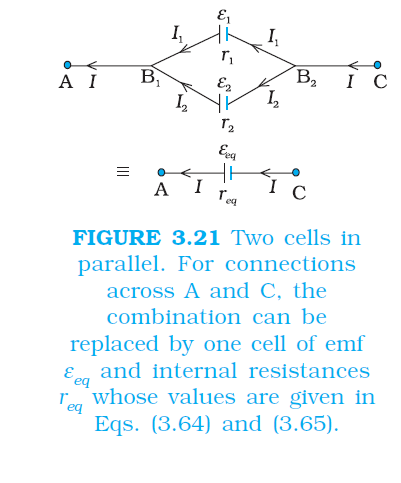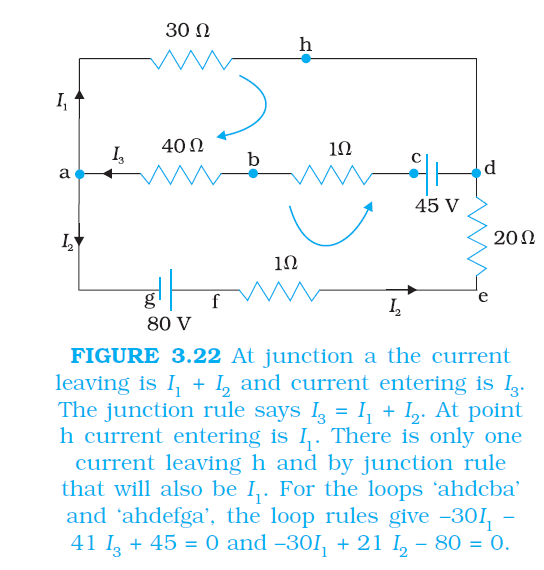Mathematics CELLS IN SERIES AND IN PARALLEL ,KIRCHHOFF’S RULES FOR CBSE-NCERT-5

### Topic covered

color{blue}{star} CELLS IN SERIES AND IN PARALLEL
color{blue}{star} KIRCHHOFF’S RULES

### CELLS IN SERIES AND IN PARALLELcolor{blue} ✍️ Like resistors, cells can be combined together in an electric circuit. And like resistors, one can, for calculating currents and voltages in a circuit, replace a combination of cells by an equivalent cell.

color{blue} ✍️ Consider first two cells in series (Fig. 3.20), where one terminal of the two cells is joined together leaving the other terminal in either cell free. ε_1, ε_2 are the emf’s of the two cells and r_1, r_2 their internal resistances, respectively. Let V (A), V (B), V (C) be the potentials at points A, B and C shown in Fig. 3.20.color{blue} ✍️ Then V (A) – V (B) is the potential difference between the positive and negative terminals of the first cell. We have already calculated it in Eq. (3.57) and hence,

color{purple}{V_(AB) ≡V(A) –V(B) =ε_1 – I r_1}

.........(3.60)

Similarly,

color{purple}{V_(BC) ≡V (B) – V (C)=ε_2 – I r_2}

..........(3.61)

color{blue} ✍️ Hence, the potential difference between the terminals A and C of the combination

V ≡ V(A) – V(C)= [V(A) - V(B) ] + [V (B) - V(C)]

color{purple}{= (ε_1+ε_2)-I (r_1+r_2)}

.........(3.62)

color{blue} ✍️ If we wish to replace the combination by a single cell between A and C of emf ε_(eq) and internal resistance r_(eq), we would have

color{purple}{V_(AC) = ε_(eq)– I r_(eq)}

.............(3.63)

color {blue}{➢➢}Comparing the last two equations, we get

color{purple}{ε_(eq) = ε_1 + ε_2}

.............(3.64)

and

color{purple}{r_(eq) = r_1 + r_2}

..............(3.65)

color{blue} ✍️ In Fig.3.20, we had connected the negative electrode of the first to the positive electrode of the second. If instead we connect the two negatives, Eq. (3.61) would change to V_(BC) = –ε_2–Ir_2 and we will get

color{purple}{ε_(eq) = ε_1 – ε_2 (ε_1 > ε_2)}

...........(3.66)

color{blue} ✍️ The rule for series combination clearly can be extended to any number of cells:
color{blue} {(i) }The equivalent emf of a series combination of n cells is just the sum of their individual emf’s, and
color{blue} { (ii)} The equivalent internal resistance of a series combination of n cells is just the sum of their internal resistances.

color{blue} ✍️ This is so, when the current leaves each cell from the positive electrode. If in the combination, the current leaves any cell from the negative electrode, the emf of the cell enters the expression for ε_eq with a negative sign, as in Eq. (3.66).

color{blue} ✍️ Next, consider a parallel combination of the cells (Fig. 3.21). I_1 and I_2 are the currents leaving the positive electrodes of the cells. At the point B_1, I_1  and I_2 flow in whereas the current I flows out. Since as much charge flows in as out, we have

color{purple}{I = I_1 + I_2}

.............(3.67)

color{blue} ✍️ Let V (B_1) and V (B_2) be the potentials at B_1 and B_2, respectively.

color{blue} ✍️ Then, considering the first cell, the potential difference across its terminals is V (B_1) – V (B_2). Hence, from Eq. (3.5)

color{purple}{V ≡V (B_1 ) – V (B_2 )=ε_1 - I_1 r_1}

...............(3.68)

color{blue} ✍️ Points B_1 and B_2 are connected exactly similarly to the second cell. Hence considering the second cell, we also have

color{purple}{V ≡V B –V B_2 =ε_2 – I_2 r_2}

............(3.69)

color {blue}{➢➢}Combining the last three equations

I = I_1 + I_2

color{blue}{= (ε_1-V)/(r_1) + (ε_2-V)/(r_2) = ((ε_1)/r_1) + (ε_2)/(r_2) - V (1/(r_2) + 1/(r_2))}

..............(3.70)

color {blue}{➢➢} Hence, V is given by,

color{purple}{V = (ε_1r_2+ε_2r_1)/(r_1+r_2) - I(r_1r_2)/(r_1+r_2)}

............(3.71)

color{blue} ✍️ If we want to replace the combination by a single cell, between B_1 and B_2, of emf ε_eq and internal resistance r_eq, we would have

color{purple}{V = ε_(eq) - I r_(eq)}

...........(3.72)

color{blue} ✍️ The last two equations should be the same and hence

color{purple}{V = (ε_1r_2+ε_2r_1)/(r_1+r_2)}

............(3.73)

color{purple}{r_(eq)= (r_1r_2)/(r_1+r_2)}

.............(3.74)

color{blue} ✍️ We can put these equations in a simpler way,

color{purple}{1/(r_(eq)) = 1/(r_1+1/(r_2)}

.............(3.75)

color{purple}{ε_(eq)/(r_(eq)) = (ε_1)/(r_1) + (ε_2)/(r_2)}

............(3.76)

color{blue} ✍️ In Fig. (3.21), we had joined the positive terminals together and similarly the two negative ones, so that the currents I_1, I_2 flow out of positive terminals.

color{blue} ✍️ If the negative terminal of the second is connected to positive terminal of the first, Eqs. (3.75) and (3.76) would still be valid with ε_2 → –ε_2 Equations (3.75) and (3.76) can be extended easily. If there an n cells of emf ε_1, . . . ε_n and of internal resistances r_1, . . . r_n respectively, connected in parallel, the combination is equivalent to a single cell of emf ε_(eq) and internal resistance r_(eq), such that

color{purple}{1/(r_(eq)) = 1/(r_1) + ....... + 1/(r_n)}

............(3.77)

color{purple}{(ε_(eq))/(r_(eq)) = (ε_1)/(r_1) + ...+ (ε_n)/(r_n)}

...........(3.78)

### KIRCHHOFF’S RULEScolor{blue} ✍️ Electric circuits generally consist of a number of resistors and cells interconnected sometimes in a complicated way. The formulae we have derived earlier for series and parallel combinations of resistors are not always sufficient to determine all the currents and potential differences in the circuit.

color{blue} ✍️ Two rules, called Kirchhoff’s rules, are very useful for analysis of electric circuits. Given a circuit, we start by labelling currents in each resistor by a symbol, say I, and a directed arrow to indicate that a current I flows along the resistor in the direction indicated.

color{blue} ✍️ If ultimately I is determined to be positive, the actual current in the resistor is in the direction of the arrow. If I turns out to be negative, the current actually flows in a direction opposite to the arrow.

color{blue} ✍️ Similarly, for each source (i.e., cell or some other source of electrical power) the positive and negative electrodes are labelled as well as a directed arrow with a symbol for the current flowing through the cell.

color{blue} ✍️ This will tell us the potential difference, V = V (P) – V (N) = ε – I r [Eq. (3.57) between the positive terminal P and the negative terminal N; I here is the current flowing from N to P through the cell].

color{blue} ✍️ If, while labelling the current I through the cell one goes from P to N, then of course

color{purple}{V = ε + I r}

...........(3.79)

color {blue}{➢➢} Having clarified labelling, we now state the rules and the proof: (a) Junction rule: At any junction, the sum of the currents entering the junction is equal to the sum of currents leaving the junction (Fig. 3.22).

color{blue} ✍️ This applies equally well if instead of a junction of several lines, we consider a point in a line. The proof of this rule follows from the fact that when currents are steady, there is no accumulation of charges at any junction or at any point in a line.

color{blue} ✍️ Thus, the total current flowing in, (which is the rate at which charge flows into the junction), must equal the total current flowing out. (b) Loop rule:

color{blue} ✍️ The algebraic sum of changes in potential around any closed loop involving resistors and cells in the loop is zero (Fig. 3.22). This rule is also obvious, since electric potential is dependent on the location of the point.

color{blue} ✍️ Thus starting with any point if we come back to the same point, the total change must be zero. In a closed loop, we do come back to the starting point and hence the rule.
Q 3114191950A battery of 10 V and negligible internal resistance is connected across the diagonally opposite corners of a cubical network consisting of 12 resistors each of resistance 1 Ω (Fig. 3.23). Determine the equivalent resistance of the network and the current along each edge of the cube.
Class 12 Chapter example 6Solution:

The network is not reducible to a simple series and parallel combinations of resistors. There is, however, a clear symmetry in the problem which we can exploit to obtain the equivalent resistance of the network. The paths AA′, AD and AB are obviously symmetrically placed in the network. Thus, the current in each must be the same, say, I. Further, at the corners A′, B and D, the incoming current I must split equally into the two outgoing branches. In this manner, the current in all the 12 edges of the cube are easily written down in terms of I, using Kirchhoff’s first rule and the symmetry in the problem. Next take a closed loop, say, ABCC′EA, and apply Kirchhoff’s second rule:

–IR – (1/2)IR – IR + ε = 0
where R is the resistance of each edge and ε the emf of battery. Thus,

ε =5/2 I R

The equivalent resistance R_(eq) of the network is

R_(eq) = ε/(3I) = 5/6R

For R = 1 Ω, R_(eq) = (5//6) Ω and for ε = 10 V, the total current (= 3I ) in the network is

3I = 10 V/(5//6) Ω = 12 A, i.e., I = 4 A
The current flowing in each edge can now be read off from the Fig. 3.23.

### Note :

color{blue} ✍️ It should be noted that because of the symmetry of the network, the great power of Kirchhoff’s rules has not been very apparent in Example 3.6.

color{blue} ✍️ In a general network, there will be no such simplification due to symmetry, and only by application of Kirchhoff’s rules to junctions and closed loops (as many as necessary to solve the unknowns in the network) can we handle the problem. This will be illustrated in Example 3.7.
Q 3154191954Determine the current in each branch of the network shown in Fig. 3.24.
Class 12 Chapter example 7Solution:

Each branch of the network is assigned an unknown current to be determined by the application of Kirchhoff’s rules. To reduce the number of unknowns at the outset, the first rule of Kirchhoff is used at every junction to assign the unknown current in each branch. We then have three unknowns I_1, I_2 and I_3 which can be found by applying the second rule of Kirchhoff to three different closed loops. Kirchhoff’s second rule for the closed loop ADCA gives,

10 – 4(I_1– I_2) + 2(I_2 + I_3 – I_1) – I_1 = 0
that is, 7I_1– 6I_2 – 2I_3 = 10
For the closed loop ABCA, we get
10 – 4I_2– 2 (I_2 + I_3) – I_1 = 0
that is, I_1 + 6I_2 + 2I_3 =10

For the closed loop BCDEB, we get
5 – 2 (I_2 + I_3) – 2 (I_2 + I_3 – I_1) = 0
that is, 2I_1 – 4I_2 – 4I_3 = –5
Equations (3.80 a, b, c) are three simultaneous equations in three unknowns. These can be solved by the usual method to give

I_1 = 2.5A, I_2 = 5/8 A . I_3 = 1 7/8 A

The currents in the various branches of the network are

AB: 5/8 A, \ \ CA 2 1/2 A; \ \ DEB : 1 7/8 A

AD 1 7/8 A; \ \ CD:0A; \ \ BC : 21/2A

It is easily verified that Kirchhoff’s second rule applied to the remaining closed loops does not provide any additional independent equation, that is, the above values of currents satisfy the second rule for every closed loop of the network. For example, the total voltage drop over the closed loop BADEB

5V+(5/8xx4) V- (15/8xx4)
equal to zero, as required by Kirchhoff’s second rule.